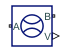# Flow Rate Sensor (IL)

Measure mass flow rate and volumetric flow rate

• Library:
• Simscape / Foundation Library / Isothermal Liquid / Sensors

•## Description

The Flow Rate Sensor (IL) block represents an ideal sensor that measures mass flow rate and volumetric flow rate in an isothermal liquid network. There is no change in pressure or temperature across the sensor.

Because the flow rate is a Through variable, the block must connect in series with the component being measured.

Ports A and B represent the sensor inlet and outlet. The relative orientation of ports A and B establishes the measurement sign. The sign is positive if flow occurs from port A to port B. Switching port connections reverses the measurement sign.

Physical signal ports M and V output the mass flow rate and the volumetric flow rate through the sensor, respectively. The Sensor Measurements parameter controls the measurement type by exposing or hiding the respective ports. Connect an exposed physical signal port to a PS-Simulink Converter block to transform the output physical signal into a Simulink® signal, for example, for plotting or additional data processing.

The block icon changes depending on the value of the Sensor Measurements parameter.

Sensor MeasurementsBlock Icon
`Mass flow rate``Volumetric flow rate``Mass and volumetric flow rates`If the sensor measurements include volumetric flow rate, the block calculates it from the measured mass flow rate:

`$q=\frac{\stackrel{˙}{m}}{\rho }$`

where:

• q is the volumetric flow rate.

• $\stackrel{˙}{m}$ is the measured mass flow rate.

• ρ is fluid mixture density at port A or B. There is no pressure difference across the sensor, therefore the densities at ports A and B are identical. Equations used to compute the fluid mixture density depend on the selected isothermal liquid model. For detailed information, see Isothermal Liquid Modeling Options.

## Ports

### Output

expand all

Physical signal output port for the mass flow rate measurement.

#### Dependencies

This port is visible if you set the Sensor Measurements parameter to `Mass flow rate` or ```Mass and volumetric flow rate```.

Physical signal output port for the volumetric flow rate measurement.

#### Dependencies

This port is visible if you set the Sensor Measurements parameter to `Volumetric flow rate` or ```Mass and volumetric flow rate```.

### Conserving

expand all

Isothermal liquid conserving port. Flow rate is positive if fluid flows from port A to port B.

Isothermal liquid conserving port. Flow rate is positive if fluid flows from port A to port B.

## Parameters

expand all

Select the type of measurements:

• `Mass flow rate` — The sensors measures the mass flow rate. Selecting this option exposes the output port M.

• `Volumetric flow rate` — The sensors measures the volumetric flow rate. Selecting this option exposes the output port V.

• `Mass and volumetric flow rate` — The sensors measures both the mass flow rate and the volumetric flow rate. Selecting this option exposes the output ports M and V.

## Version History

Introduced in R2020a